Courses

# Test: Frequency Response

## 20 Questions MCQ Test Analog Circuits | Test: Frequency Response

Description
This mock test of Test: Frequency Response for Electronics and Communication Engineering (ECE) helps you for every Electronics and Communication Engineering (ECE) entrance exam. This contains 20 Multiple Choice Questions for Electronics and Communication Engineering (ECE) Test: Frequency Response (mcq) to study with solutions a complete question bank. The solved questions answers in this Test: Frequency Response quiz give you a good mix of easy questions and tough questions. Electronics and Communication Engineering (ECE) students definitely take this Test: Frequency Response exercise for a better result in the exam. You can find other Test: Frequency Response extra questions, long questions & short questions for Electronics and Communication Engineering (ECE) on EduRev as well by searching above.
QUESTION: 1

### (Q.1-Q.3) A parallel resonant circuit has a resistance of 2k ohm and half power frequencies of 86 kHz and 90 kHz. Q. The value of capacitor is

Solution:

BW = 2p(90-86)k = 1/RC or C = 19.89 nF.

QUESTION: 2

### The value of inductor is

Solution:

w = (86 + 90)k/2 = 88 = (1/LC)(0.5) or L = 0.16 mH.

QUESTION: 3

### The quality factor is

Solution:

Q = w/BW = 176pK/8pk = 22.

QUESTION: 4

(Q.4-Q.5) A parallel resonant circuit has a midband admittance of 25 X 10(-3) S, quality factor of 80 and a resonant frequency of 200 krad s.4. The value of R (in ohm) is

Solution:

At mid-band frequency Y = 1/R or R = 1000/25 or 40 ohm.

QUESTION: 5

The value of C is

Solution:

Q = wRC or C = 80/(200 x 1000 x 40) or 10 µF.

QUESTION: 6

A parallel RLC circuit has R 1 k and C 1 F. The quality factor at resonance is 200. The value of inductor is

Solution:

Use Q = R (L/C)0.5.

QUESTION: 7

A parallel circuit has R = 1k ohm , C = 50 µF and L = 10mH. The quality factor at resonance is

Solution:

Use Q = R (L/C)0.5.

QUESTION: 8

A series resonant circuit has L = 1 mH and C = 10 F. The required R (in ohm) for the BW = 15 9 . Hz is

Solution:

Use BW = R/L.

QUESTION: 9

For the RLC parallel resonant circuit when R = 8k, L = 40 mH and C = 0.25 F, the quality factorQ is

Solution:

use Q = R (C/L)0.5.

QUESTION: 10

A series resonant circuit has an inductor L = 10 mH. The resonant frequency w = 10^6 rad/s and bandwidth is BW = 103 rad/s. The value of R and C will be

Solution:

Use wxw = 1/LC and BW = R/L.

QUESTION: 11

The maximum voltage across capacitor would be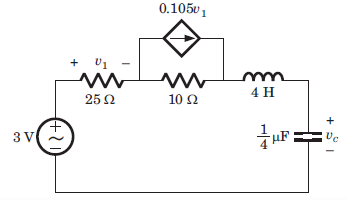Solution:

Explanation : LC combination

3 = v1 + 10(v1 - 0.105v1)/125

= v1 = 100

Isc = 1100/125

= 0.8V

RTH = 3/0.8

= 3.75 ohm

Wo = 1/(LC)1/2

= 1000

Q = w0L/RTH

= (1000*4)/3.75

= 1066.67

|vc|max = Q*vTH

= 1066.67*3

= 3200V

QUESTION: 12

Find the resonnant frequency for the circuit.​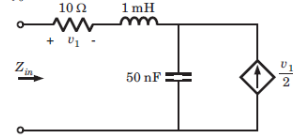Solution: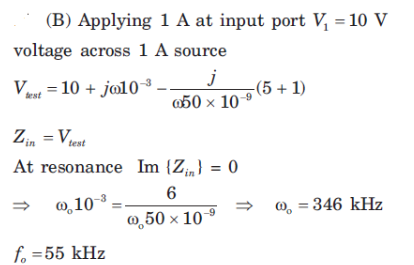QUESTION: 13

Determine the resonant frequency of the circuit.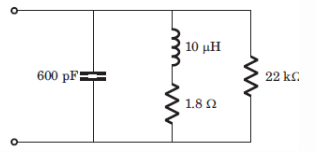Solution: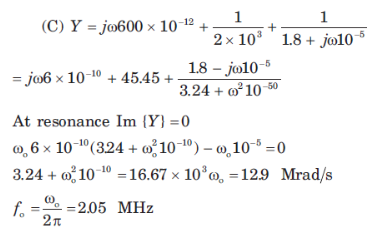QUESTION: 14

The value of C and A for the given network function is​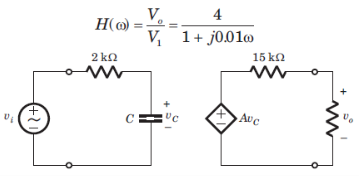Solution: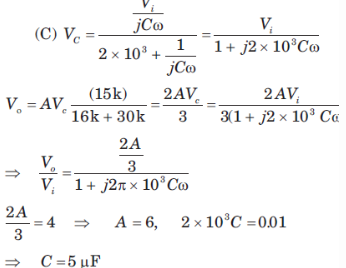QUESTION: 15

H(w) = Vo/Vi = ?​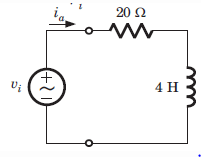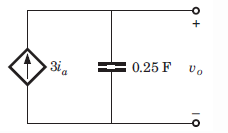Solution: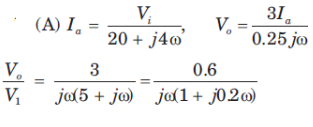QUESTION: 16

H(w) = Vo/Vi = ?​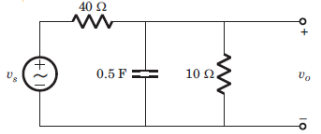Solution: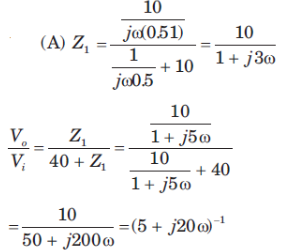QUESTION: 17

The value of input frequency is required to cause a gain equal to 1.5. The value is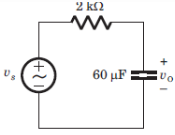Solution: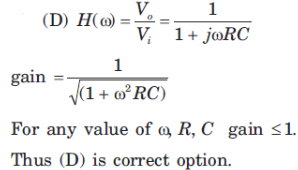QUESTION: 18

In the circuit shown phase shift equal to 45 degrees and is required at frequency w = 20 rad/s. The value of R (in kilo-ohm) is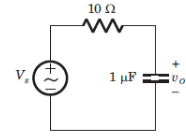Solution: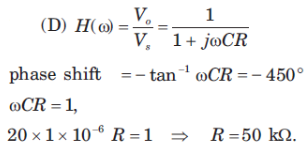QUESTION: 19

For the circuit shown the input frequency is adjusted until the gain is equal to 0.6. The value of the frequency is​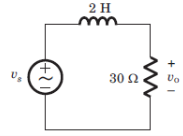Solution: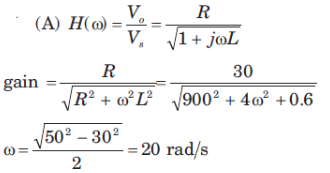QUESTION: 20

For a stable closed loop system, the gain at phase crossover frequency should always be:

Solution:

Phase crossover frequency is the frequency at which the gain of the system must be 1 and for a stable system the gain is decibels must be 0 db.